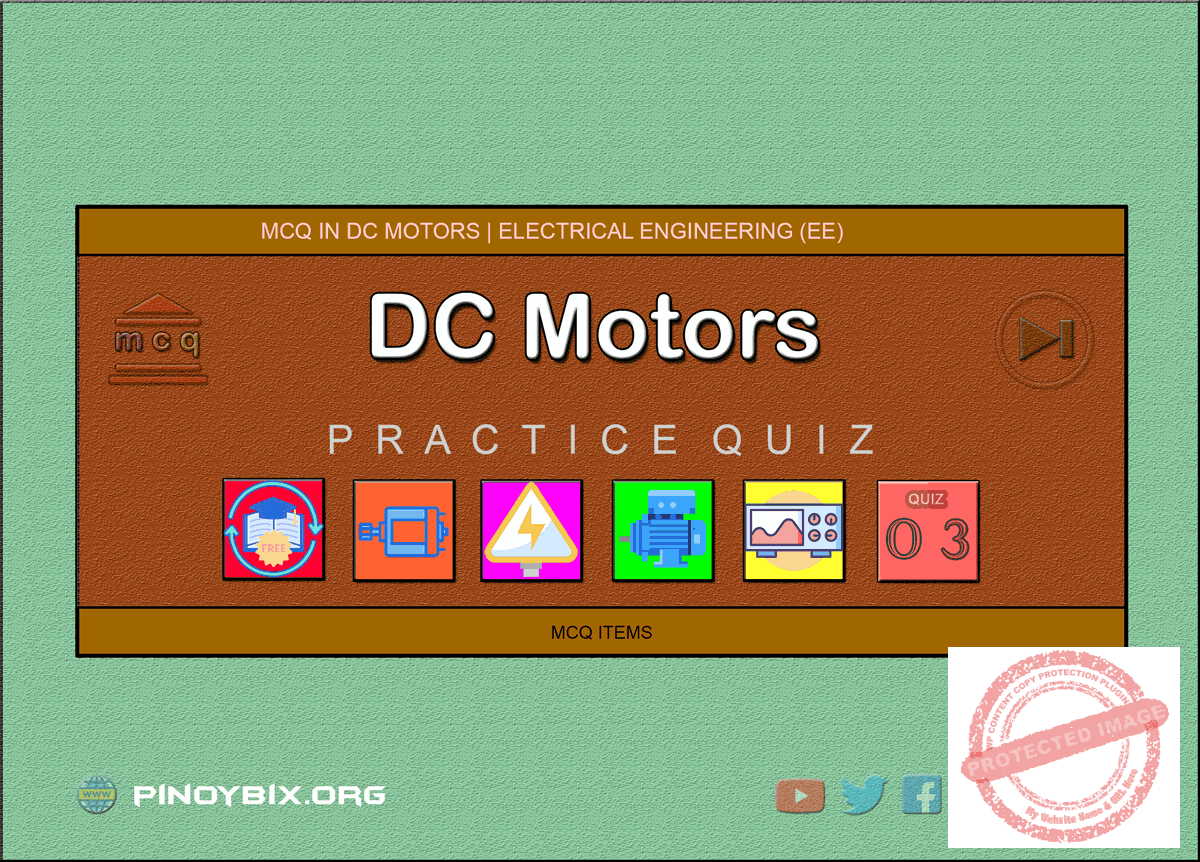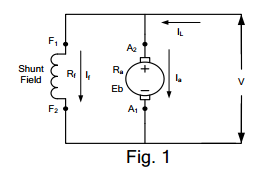# MCQ in DC Motors Part 3 | REE Board Exam

(Last Updated On: July 28, 2020)This is the Multiple Choice Questions Part 3 of the Series in DC Motors as one of the Electrical Engineering topic. In Preparation for the REE Board Exam make sure to expose yourself and familiarize in each and every questions compiled here taken from various sources including but not limited to past Board Exam Questions in Electrical Engineering field, Electrical Engineering Books, Journals and other Electrical Engineering References.

#### Continue Practice Exam Test Questions Part 3 of the Series

Choose the letter of the best answer in each questions.

101.  In Fig. 1, the quantity VIa representsA.  mechanical output of motor

B.  core losses

C.  friction and windage losses

D.  all losses

Solution:

102.  The mechanical power developed in a dc motor is maximum when back emf (Eb) is equal to ____ the applied voltage (V).

A.  twice

B.  half

C.  one-third

D.  none of these

Solution:

103.  When the speed of a dc shunt motor increases, its armature current

A.  increases

B.  decreases

C.  remains constant

D.  none of these

Solution:

104.  The amount of back emf of a shunt motor will increase when

B.  the field is weakened

C.  the field is strengthened

D.  none of these

Solution:

105.  The speed of a dc motor is

A.  directly proportional to flux per pole

B.  inversely proportional to flux per pole

C.   inversely proportional to applied voltage

D.  none of these

Solution:

106.  The torque developed by a dc motor is directly proportional to

A.  flux per pole x armature current

B.  armature resistance x applied voltage

C.  armature resistance x armature current

D.  none of these

Solution:

107.  The shaft torque (Tsh) in a dc motor is less than total armature torque (Ta) because of

A.  Cu losses

B.  field losses

C.  iron and friction losses

D.  none of these

Solution:

108.  Armature reaction in a dc motor is increased

A.  when the armature current increases

B.  when the armature current decreases

C.  when the field current decreases

D.  by interpoles

Solution:

109.  With respect to the direction of rotation, interpoles on a dc motor must have the same polarity as the main poles

B.  behind them

C.  along them

C.  none of these

Solution:

110.  In a dc motor, the brushes are shifted from the mechanical neutral plane in direction opposite to the rotation to

A.  decrease speed

B.  increase speed

C.  reduce sparking

D.  produce flat characteristics

Solution:

111.  In very large dc motors with severe heavy duty, armature reaction effects are corrected by

A.  using interpoles only

B.  using compensatory windings in addition to interpoles

C.  shifting the brush position

D.  none of these

Solution:

112.  The speed of a ____ motor is practically constant.

A.  cummulatively compounded

B.  series

C.  differentially compounded

D.  shunt

Solution:

113.  ____ motor is a variable speed motor.

A.  series

B.  shunt

C.  cummulatively compounded

D.  differentially compounded

Solution:

114.  The most commonly used method of speed control of a dc motor is by varying

A.  voltage applied to the motor

B.  field strength

C.  effective number of conductors in series

D.  armature circuit resistance

Solution:

115.  The running speed of a dc series motor is basically determined by

A.  field excitation

C.  armature resistance

D.  none of these

Solution:

116.  After a shunt motor is up to speed, the speed may be increased considerably by

A.  increasing field circuit resistance

B.  decreasing field circuit resistance

C.  increasing armature circuit resistance

Solution:

117.  ____ motor has the best speed regulation.

A.  series

B.  cummulatively compounded

C.  shunt motor

D.  differentially compounded

Solution:

118.  The deciding factor in the selection of a dc motor for a particular application is its ______ characteristics.

A.  speed-torque

B.  speed-armature current

C.  torque-armature current

D.  none of these

Solution:

119.  The demand for a large increase in torque of a dc shunt motor is met by a

A.  large decrease in speed

B.  large increase in speed

C.  large increase in current

D.  small increase in current

Solution:

120.  The demand for a large increase in torque of a dc series motor is met by a

A.  large decrease in current

B.  large decrease in speed

C.  large increase in speed

D.  small decrease in speed

Solution:

121.  A series motor will over-speed when

B.  the field is opened

C.  the armature circuit is opened

Solution:

122.  As the load increases, a ____ motor will speed up.

A.  series

B.  shunt

C.  cummulatively compounded

D.  differentially compounded

Solution:

123.  A cummulatively compounded motor does not run at dangerous speed at light loads because of the presence of

A.  shunt winding

B.  series winding

C.  interpoles

D.  compensating winding

Solution:

124.  DC shunt motors are used in applications where ____ is required.

A.  high starting torque

B.  practically constant speed

D.  variable speed

Solution:

125.  DC series motors are used in applications where ______ is required.

A.  high starting torque

B.  constant speed

D.  none of these

Solution:

126.  Cummulatively compounded motors are used where we require

A.  variable speed

B.  poor speed regulation

C.  sudden heavy loads for short duration

D.  none of these

Solution:

127.  For the same rating, ____ motor has the highest starting torque.

A.  shunt

B.  cummulatively compounded

C.  differentially compounded

D.  series

Solution:

128.  _____ motors never use belt-connected loads.

A.  series

B.  shunt

C.  cummulatively compounded

D.  differentially compounded

Solution:

129.  For 20% increase in current, the motor that will give the greatest increase in torque is ____ motor.

A.  shunt

B.  series

C.  cummulatively compounded

D.  differentially compounded

Solution:

130.  The ____ motor is used to start heavy loads.

A.  series

B.  shunt

C.  differentially compounded

D.  none of these

Solution:

131.  When load is removed, the motor that will run at the highest speed is

A.  shunt

B.  series

C.  cummulatively compounded

D.  differentially compounded

Solution:

132.  For the same rating, ____ motor has the least starting torque.

A.  cummulatively compounded

B.  series

C.  shunt

D. none of these

Solution:

133.  A dc series motor is most suitable for

A.  cranes

B.  pump

C.  lathes

D.  punch presses

Solution:

134.  ____ is most suitable for punch presses.

A.  shunt

B.  series

C.  differentially compounded

D.  cummulatively compounded

Solution:

135.  In a vacuum cleaner, we generally used ____ motor.

A.  shunt

B.  cummulatively compounded

C.  series

D.  differentially compounded

Solution:

136.  The most suitable motor for elevators is the ____ motor.

A.  series

B.  shunt

C.  differentially compounded

D.  cummulatively compounded

Solution:

137.  The friction and windage losses in a dc motor depends upon

A.  speed

B.  flux

C.  armature current

D.  field and armature resistance

Solution:

138.  The iron losses in a dc motor depend upon

A.  flux only

B.  speed only

C.  both speed and flux

D.  none of these

Solution:

139.  The greatest percentage of power loss in a dc motor is due to

A.  windage loss

B.  copper loss

C.  core loss

D.  friction loss

Solution:

140.  A motor takes a large current at starting because

A.  the armature resistance is high

B.  back emf is low

C.  shunt field is producing weak field

D.  none of these

Solution:

141.  Excessive sparking at the brushes may be cause due to

A.  dirt on the commutator

B.  loose coupling

C.  misalignment of machine

D.  worn bearings

Solution:

142.  Excessive motor vibration is caused by

A.  too much brush tension

B.  worn bearings

C.  open armature coil

D.  bent shaft

Solution:

143.  Overheating of a dc motor is often due to

A.  insufficient end play

B.  loose parts

D.  rough commutator

Solution:

144.  Hot bearings of a dc motor may be caused by

A.  poor ventilation

B.  incorrect voltage

C.  loose coupling

D.  lack or dirty lubricant

Solution:

145.  Intermittent sparking at the brushes of a dc motor may be caused due to

A.  an open armature coil

C.  loose coupling

D.  incorrect voltage

Solution:

146.  The function of a brush and commutator arrangement in a dc motor is

A.  to produce unidirectional torque

B.  to produce unidirectional current in the armature

C.  to help changing the direction of rotation of the armature

D.  to reduce sparking

Solution:

147.  The direction of rotation of a dc motor can be reversed

A.  by reversing the connections of both armature and the field windings with the supply

B.  by reversing the connections of both armature or the filed winding connections with the supply

C.  by reducing the field flux

D.  by introducing an extra resistance in the armature circuit

Solution:

148.  A dc series motor should always be started with load because

A.  at no-load it will rotate at a dangerously high speed

B.  at no-load it will not develop high starting torque

D.  it draws small amount of current at no-load

Solution:

149.  Swinburne’s method cannot be used for determining the efficiency of a dc series motor because

A.  it is not advisable to run a series motor on no-load

B.  a series motor takes excessive current at no-load

C.  a series motor develops very high starting torque

D.  it is not possible to load a series motor in steps

Solution:

150.  What is the effect produced by the electric motor?

A.  magnetic effect only

B.  magnetic as well as heating effect

C.  heating effect only

D.  heating as well as chemical effect

Solution:

P inoyBIX educates thousands of reviewers and students a day in preparation for their board examinations. Also provides professionals with materials for their lectures and practice exams. Help me go forward with the same spirit.

“Will you subscribe today via YOUTUBE?”

Subscribe

PinoyBIX Engineering. © 2014-2020 All Rights Reserved | How to Donate? |